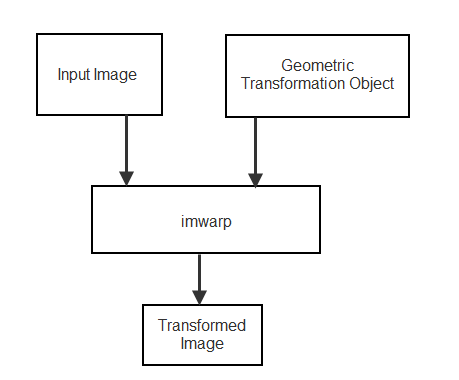## 2-D and 3-D Geometric Transformation Process Overview

To perform a 2-D or 3-D geometric transformation, first create a geometric transformation object that stores information about the transformation. Then, pass the image to be transformed and the geometric transformation object to the `imwarp` function. You optionally can provide spatial referencing information about the input image to `imwarp`.`imwarp` uses the geometric transformation to map coordinates in the output image to the corresponding coordinates in the input image (inverse mapping). Then, `imwarp` uses the coordinate mapping to interpolate pixel values within the input image and calculate the output pixel value.

### Create Geometric Transformation Object

Different types of geometric transformation objects store different information about the transformation.

There are several ways to create a geometric transformation object.

Approach to Create Geometric Transformation

`transltform2d`

`transltform3d`

`rigidtform2d`

`rigidtform3d`

`simtform2d`

`simtform3d`

`affinetform2d`

`affinetform3d`

`projective2d`

`geometricTransform2d`

`geometricTransform3d`

Other Geometric Transformations
Specify Translation, Rotation, or Scale ParametersXX
Specify Transformation MatrixXXXX
Specify Custom Point-Wise Mapping Function    X
Estimate Transformation from Control Point Pairs X (2-D)X (2-D)X (2-D) X
Estimate Transformation Using Similarity OptimizationX (2-D)X (2-D)X (2-D)
Estimate Transformation Using Phase CorrelationX (2-D)X (2-D)
Generate Random Affine Transformations  X

#### Specify Translation, Rotation, or Scale Parameters

If you know the amount of translation, the rotation angle, and the scale factor, then you can create a transformation by specifying these parameters.

The following example defines a translation and rotation angle, then creates a `rigidtform2d` geometric transformation object from the specified parameters.

```theta = 30; translation = [10 20.5]; tform = rigidtform2d(theta,translation)```
```tform = rigidtform2d with properties: Dimensionality: 2 RotationAngle: 30 Translation: [10 20.5000] R: [2×2 double] A: [3×3 double]```

#### Specify Transformation Matrix

For more complex linear geometric transformations, you can represent the transformation as a matrix. For example, use a matrix representation for projective transformations or for affine transformations involving reflection, anisotropic scaling, shear, or compositions of linear transformations. Specify the transformation matrix to create an `affinetform2d`, `affinetform3d`, or `projtform2d` object. For more information about creating a transformation matrix, see Matrix Representation of Geometric Transformations.

The following example defines the transformation matrix for nonisotropic scaling and reflection about the y axis, then creates an `affinetform2d` geometric transformation object from the transformation matrix.

```scaleX = 0.8; scaleY = 1.5; A = [scaleX 0 0; 0 -scaleY 0; 0 0 1]; tform = affinetform2d(A)```
```tform = affinetform2d with properties: Dimensionality: 2 A: [3×3 double]```

#### Specify Custom Point-Wise Mapping Function

If you have an inverse point-wise mapping function, then you can create a custom 2-D and 3-D geometric transformation using the `geometricTransform2d` and the `geometricTransform3d` objects respectively.

The following example specifies an inverse mapping function that accepts and returns 2-D points in packed (x,y) format. Then, the example creates a `geometricTransform2d` geometric transformation object from the inverse mapping function.

`inversefn = @(c) [c(:,1)+c(:,2),c(:,1).^2]`
```inversefn = function_handle with value: @(c)[c(:,1)+c(:,2),c(:,1).^2]```
`tform = geometricTransform2d(inversefn)`
```tform = geometricTransform2d with properties: InverseFcn: [function_handle] ForwardFcn: [] Dimensionality: 2```

Similarly, the following example creates a `geometricTransform3d` geometric transformation object using the inverse mapping function. The example specifies an inverse mapping function that accepts and returns 3-D points in packed (x,y,z) format.

`inversefn = @(c)[c(:,1)+c(:,2),c(:,1)-c(:,2),c(:,3).^2]`
```inversefn = function_handle with value: @(c)[c(:,1)+c(:,2),c(:,1)-c(:,2),c(:,3).^2]```
`tform = geometricTransform3d(inversefn)`
```tform = geometricTransform3d with properties: InverseFcn: [function_handle] ForwardFcn: [] Dimensionality: 3```

#### Estimate Transformation from Control Point Pairs

You can create a geometric transformation object by passing pairs of control points to the `fitgeotform2d` function. The `fitgeotform2d` function automatically estimates the transformation from these points and returns one of the geometric transformation objects.

Different transformations require a varying number of points. For example, affine transformations require three non-collinear points in each image (a triangle) and projective transformations require four points (a quadrilateral).

This example defines two pairs of control points, then uses the `fitgeotform2d` to create an `affinetform2d` geometric transformation object.

```movingPoints = [11 11;21 11; 21 21]; fixedPoints = [51 51;61 51;61 61]; tform = fitgeotform2d(movingPoints,fixedPoints,"affine")```
```tform = affinetform2d with properties: Dimensionality: 2 A: [3×3 double]```

#### Estimate Transformation Using Similarity Optimization

If you have a fixed image and a moving image that are slightly misaligned, then you can use the `imregtform` function to estimate an affine geometric transformation that aligns the images. `imregtform` optimizes the mean squares or Mattes mutual information similarity metric of the two images, using a regular step gradient descent or one-plus-one evolutionary optimizer. For more information, see Create an Optimizer and Metric for Intensity-Based Image Registration.

#### Estimate Transformation Using Phase Correlation

If you have a fixed image and a moving image that are severely misaligned, then you can use the `imregcorr` function to estimate an affine geometric transformation that improves the image alignment. You can refine the resulting transformation by using similarity optimization.

#### Generate Random Affine Transformations

You can create an affine geometric transformation with randomized transformation parameters using the `randomAffine2d` and `randomAffine3d` functions. These functions support all affine parameters including reflection about each axis, rotation, shearing, and nonisotropic scale factors. Randomized affine transformations are commonly used as a data augmentation technique for deep learning.